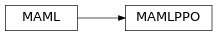# garage.torch.algos.maml_ppo¶

Model-Agnostic Meta-Learning (MAML) algorithm applied to PPO.

class MAMLPPO(env, policy, value_function, task_sampler, inner_lr=_Default(0.1), outer_lr=0.001, lr_clip_range=0.5, discount=0.99, gae_lambda=1.0, center_adv=True, positive_adv=False, policy_ent_coeff=0.0, use_softplus_entropy=False, stop_entropy_gradient=False, entropy_method='no_entropy', meta_batch_size=20, num_grad_updates=1, meta_evaluator=None, evaluate_every_n_epochs=1)Model-Agnostic Meta-Learning (MAML) applied to PPO.

Parameters
• env (Environment) – A multi-task environment.

• policy (garage.torch.policies.Policy) – Policy.

• value_function (garage.np.baselines.Baseline) – The value function.

• inner_lr (float) – Adaptation learning rate.

• outer_lr (float) – Meta policy learning rate.

• lr_clip_range (float) – The limit on the likelihood ratio between policies.

• discount (float) – Discount.

• gae_lambda (float) – Lambda used for generalized advantage estimation.

• center_adv (bool) – Whether to rescale the advantages so that they have mean 0 and standard deviation 1.

• positive_adv (bool) – Whether to shift the advantages so that they are always positive. When used in conjunction with center_adv the advantages will be standardized before shifting.

• policy_ent_coeff (float) – The coefficient of the policy entropy. Setting it to zero would mean no entropy regularization.

• use_softplus_entropy (bool) – Whether to estimate the softmax distribution of the entropy to prevent the entropy from being negative.

• entropy_method (str) – A string from: ‘max’, ‘regularized’, ‘no_entropy’. The type of entropy method to use. ‘max’ adds the dense entropy to the reward for each time step. ‘regularized’ adds the mean entropy to the surrogate objective. See https://arxiv.org/abs/1805.00909 for more details.

• meta_batch_size (int) – Number of tasks sampled per batch.

• meta_evaluator (garage.experiment.MetaEvaluator) – A meta evaluator for meta-testing. If None, don’t do meta-testing.

• evaluate_every_n_epochs (int) – Do meta-testing every this epochs.

train(self, trainer)

Obtain samples and start training for each epoch.

Parameters

trainer (Trainer) – Gives the algorithm access to :method:~Trainer.step_epochs(), which provides services such as snapshotting and sampler control.

Returns

The average return in last epoch cycle.

Return type

float

property policy(self)

Current policy of the inner algorithm.

Returns

Current policy of the inner

algorithm.

Return type

garage.torch.policies.Policy

get_exploration_policy(self)

Each time it is retrieved, this policy should only be evaluated in one task.

Returns

The policy used to obtain samples that are later used for

Return type

Policy

adapt_policy(self, exploration_policy, exploration_episodes)

Parameters
• exploration_policy (Policy) – A policy which was returned from get_exploration_policy(), and which generated exploration_episodes by interacting with an environment. The caller may not use this object after passing it into this method.

• exploration_episodes (EpisodeBatch) – Episodes with which to adapt, generated by exploration_policy exploring the environment.

Returns